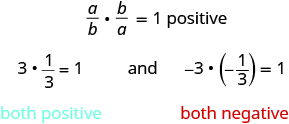Finding the Reciprocal of a Number

Learning Outcomes

• Find the reciprocal of a fraction
• Recognize the difference between absolute value, reciprocal and opposite of a fraction or number

The fractions $\Large\frac{2}{3}$ and $\Large\frac{3}{2}$ are related to each other in a special way. So are $\Large-\frac{10}{7}$ and $\Large-\frac{7}{10}$. Do you see how? Besides looking like upside-down versions of one another, if we were to multiply these pairs of fractions, the product would be $1$.

$\Large\frac{2}{3}\cdot \frac{3}{2}\normalsize=1\text{ and }-\Large\frac{10}{7}\left(-\frac{7}{10}\right)\normalsize=1$

Such pairs of numbers are called reciprocals.

Reciprocal

The reciprocal of the fraction $\Large\frac{a}{b}$ is $\Large\frac{b}{a}$, where $a\ne 0$ and $b\ne 0$,
A number and its reciprocal have a product of $1$.

$\Large\frac{a}{b}\cdot \frac{b}{a}\normalsize=1$

To find the reciprocal of a fraction, we invert the fraction. This means that we place the numerator in the denominator and the denominator in the numerator.

To get a positive result when multiplying two numbers, the numbers must have the same sign. So reciprocals must have the same sign.To find the reciprocal, keep the same sign and invert the fraction. The number zero does not have a reciprocal. Why? A number and its reciprocal multiply to $1$. Is there any number $r$ so that $0\cdot r=1?$ No. So, the number $0$ does not have a reciprocal.

Example

Find the reciprocal of each number. Then check that the product of each number and its reciprocal is $1$.

1. $\Large\frac{4}{9}$
2. $\Large-\frac{1}{6}$
3. $\Large-\frac{14}{5}$
4. $7$

Solution:
To find the reciprocals, we keep the sign and invert the fractions.

 1. Find the reciprocal of $\Large\frac{4}{9}$ The reciprocal of $\Large\frac{4}{9}$ is $\Large\frac{9}{4}$ Check: Multiply the number and its reciprocal. $\Large\frac{4}{9}\cdot \frac{9}{4}$ Multiply numerators and denominators. $\Large\frac{36}{36}$ Simplify. $1\quad\checkmark$
 2. Find the reciprocal of $\Large-\frac{1}{6}$ $\Large-\frac{6}{1}$ Simplify. $-6$ Check: $\Large-\frac{1}{6}\normalsize\cdot \left(-6\right)$ $1\quad\checkmark$
 3. Find the reciprocal of $\Large-\frac{14}{5}$ $\Large-\frac{5}{14}$ Check: $\Large-\frac{14}{5}\cdot \left(-\frac{5}{14}\right)$ $\Large\frac{70}{70}$ $1\quad\checkmark$
 4. Find the reciprocal of $7$ Write $7$ as a fraction. $\Large\frac{7}{1}$ Write the reciprocal of $\Large\frac{7}{1}$ $\Large\frac{1}{7}$ Check: $7\cdot\Large\left(\frac{1}{7}\right)$ $1\quad\checkmark$

Try It

In the following video we will show more examples of how to find the reciprocal of integers, fractions and mixed numbers.

In a previous chapter, we worked with opposites and absolute values. The table below compares opposites, absolute values, and reciprocals.

Opposite Absolute Value Reciprocal
has opposite sign is never negative has same sign, fraction inverts

Example

Fill in the chart for each fraction in the left column:

Number Opposite Absolute Value Reciprocal
$\Large-\frac{3}{8}$
$\Large\frac{1}{2}$
$\Large\frac{9}{5}$
$-5$

Try It

Fill in the chart for each number given:

Number Opposite Absolute Value Reciprocal
$\Large-\frac{5}{8}$
$\Large\frac{1}{4}$
$\Large\frac{8}{3}$
$-8$

Try it

The following video provides more examples of finding the opposite of a number.

The next video shows how to find the absolute value of an integer.